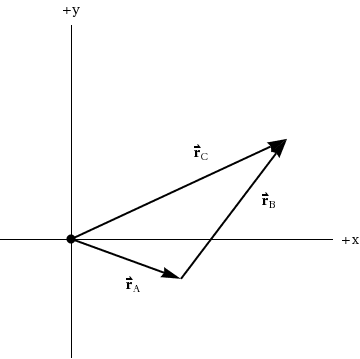Alexandra Fabiello
Homework Statement:
Two position vectors lie in a plane. The first, vector
rA,
points at an angle of 20° below the positive x-axis and has a magnitude of 55.0 m. The second, vector
rB,
points at an angle of 47.0° above the positive x-axis and has a magnitude of 75 m.

What is the magnitude and direction of vector
rC if rA + rB = rC? Give the direction as an angle measured counterclockwise from the positive x-axis.
Relevant Equations:
a^2 = b^2 + c^2 ?
not sure what else
I am admittedly entirely confused as to where to start, sorry.This is the diagram I'm given that fits with the rA + rB.

If rB is 47.0 degrees above the x-axis normally, it would be the same counter-clockwise here, right? Then 180 - 47 = 133 degrees for the clockwise angle. But now I'm stuck with how to find rC. If I assume this is actually a right angle triangle, rC ends up being about 93 degrees, but I can't really be sure it's really a right angle, so how do I do that? The book is confusing in this area, and so was the teacher.

If this is better on another thread, please let me know which one.

•Delta2

Homework Helper
Gold Member
It’s best to break up each vector into its x and y components, then add up both x components then add up both y components, and the mag of the resultant vector will then be obtained using Pythagorean’s theorem, because now you have a right triangle. The direction comes from trig. If you haven’t gone into this yet, you can use the law of cosines.

Homework Helper
Gold Member
Since I see that you have setup the triangle of the vectors, I would advise you to use the law of cosines. No it isn't a right triangle so we can't use Pythagorean, that's why we use cosine law which is a generalization of Pythagorean theorem and it holds even in non right triangles.
Now, in order to use cosine law we must know the angle between ##\vec{r_A}## and ##\vec{r_B}## (as well as the magnitudes of each vector which magnitudes are directly given by the statement). we can calculate this angle from the given data of the statement. What do you think is that angle?

After you 've found this angle and calculate the magnitude of ##\vec{r_C}##, you can then use the law of sines to calculate the angle that ##\vec{r_C}## makes with ##\vec{r_A}##.

Last edited:
Alexandra Fabiello
Well that didn't work. Do I consider the A vectors as negative when I add the components?

Homework Helper
Gold Member
Well that didn't work. Do I consider the A vectors as negative when I add the components?
You have to show us your work in order for us to see what mistakes you did. How did you applied cosines law? I suspect you used the wrong angle...

if you did it using the x and y components approach, the y component for vector A will be negative.

Homework Helper
Gold Member
Well that didn't work. Do I consider the A vectors as negative when I add the components?
The x component of the A vector is positive since it projects rightward along the positive x axis. The y component of the A vector is negative since it projects downward along the negative y axis.
You should note that both components of the B vector are positive since the x component of B projects rightward and the y component of B projects upward.

Homework Helper
Gold Member
2022 Award
Homework Statement: Two position vectors lie in a plane. The first, vector
rA,
points at an angle of 20° below the positive x-axis and has a magnitude of 55.0 m. The second, vector
rB,
points at an angle of 47.0° above the positive x-axis and has a magnitude of 75 m.

What is the magnitude and direction of vector
rC if rA + rB = rC? Give the direction as an angle measured counterclockwise from the positive x-axis.
Homework Equations: a^2 = b^2 + c^2 ?
not sure what else

I am admittedly entirely confused as to where to start, sorry.
View attachment 250660 This is the diagram I'm given that fits with the rA + rB.

If rB is 47.0 degrees above the x-axis normally, it would be the same counter-clockwise here, right? Then 180 - 47 = 133 degrees for the clockwise angle. But now I'm stuck with how to find rC. If I assume this is actually a right angle triangle, rC ends up being about 93 degrees, but I can't really be sure it's really a right angle, so how do I do that? The book is confusing in this area, and so was the teacher.

If this is better on another thread, please let me know which one.
Your diagram looks good. Note that when you add two vectors like this, you should imagine that the origin moves to the tip of the first vector. That's what tells you how to draw the second vector.

I would draw some dotted lines at the tip of the first vector to represent the translated x and y axes, then draw the second vector according to its definition.

As mentioned above, you will not always get a right angled triangle.

You can get the magnitude of the resultant vector from the law of cosine. And you should be able to get its angle from the law of sines. Alternatively using vector components is a general and reliable way to tackle the problem. The diagram should help you check you are adding the components correctly and help you check your answer looks sensible.

Alexandra Fabiello
Never mind, I got it. Just had to do a lot of diagrams and turn everything into right angle triangles, and it worked out.

Thanks everyone!